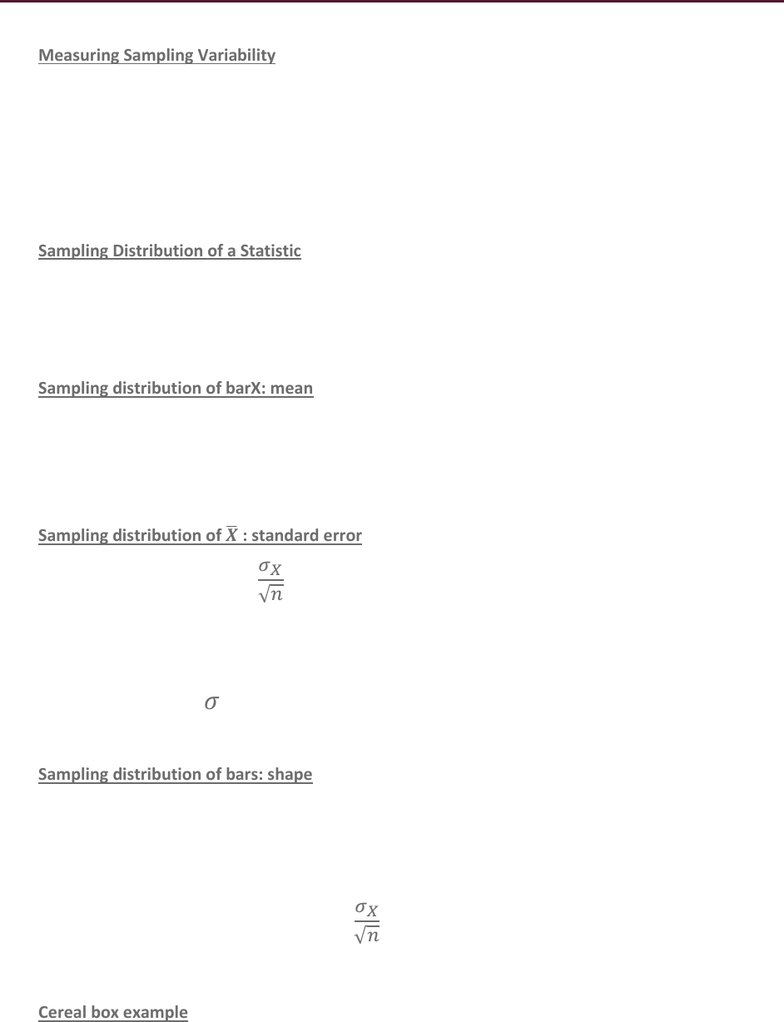# STAT 1430 Lecture Notes - Lecture 23: Statistic, Sampling Distribution, Statistical Parameter

59 views4 pagesSTAT1430.01Lecture23Binomial Distribution March 26, 2019
Measuring Sampling Variability
o Find the distribution of all possible values of the sample statistic (from all possible
samples of size n)
o This is called the: Sampling Distribution
o Find its center (mean), spread (standard error) and shape
o Use this information to make inferences about the population parameter
Sampling Distribution of a Statistic
o Take all possible samples size n from the population
o Find the statistic you need from each sample (i.e.
)
o Put all these sample statistics together.
Sampling distribution of barX: mean
o
= and possible sample mean (from many samples of size n many possible
)
o
has its own average,
known :
 
o Mean of all sample means = mean of the population
Sampling distribution of
: standard error
o Formula:
o What affects the standard error and how?
As n increases decreases more precision in
means have less variability
As increases also increases
As diversity/variability in the population increases, variability in
increases also
Sampling distribution of bars: shape
o Case 1:X has a Normal distribution with mean and standard deviation
o The shape of the distribution of X is: normal
o The shape of the sampling distribution of barX is: normal
Smaller standard error
Same mean
 
Cereal box example
Unlock document

This preview shows page 1 of the document.
Unlock all 4 pages and 3 million more documents.

## Get access

\$10 USD/m
Billed \$120 USD annuallyHomework Help
Study Guides
Textbook Solutions
Class Notes
Textbook Notes
Booster Class